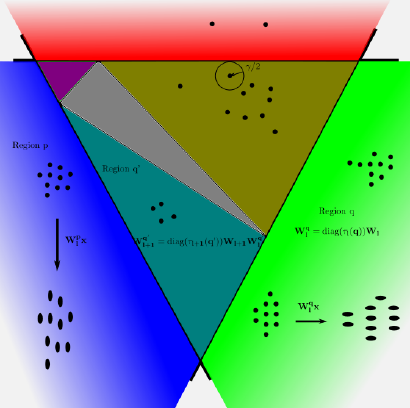# Orthogonal Deep Neural Networks### Abstract

In this paper, we introduce the algorithms of Orthogonal Deep Neural Networks (OrthDNNs) to connect with recent interest of spectrally regularized deep learning methods. OrthDNNs are theoretically motivated by generalization analysis of modern DNNs, with the aim to find solution properties of network weights that guarantee better generalization. To this end, we first prove that DNNs are of local isometry on data distributions of practical interest; by using a new covering of the sample space and introducing the local isometry property of DNNs into generalization analysis, we establish a new generalization error bound that is both scale- and range-sensitive to singular value spectrum of each of networks’ weight matrices. We prove that the optimal bound w.r.t. the degree of isometry is attained when each weight matrix has a spectrum of equal singular values, among which orthogonal weight matrix or a non-square one with orthonormal rows or columns is the most straightforward choice, suggesting the algorithms of OrthDNNs. We present both algorithms of strict and approximate OrthDNNs, and for the later ones we propose a simple yet effective algorithm called Singular Value Bounding (SVB), which performs as well as strict OrthDNNs, but at a much lower computational cost. We also propose Bounded Batch Normalization (BBN) to make compatible use of batch normalization with OrthDNNs. We conduct extensive comparative studies by using modern architectures on benchmark image classification. Experiments show the efficacy of OrthDNNs.

Publication
IEEE Transactions on Pattern Analysis and Machine Intelligence##### Yuxin Wen
###### Researcher

My research interests are in computer vision (to non-Euclidean data) and machine learning, with focus on digital human animation and modeling.